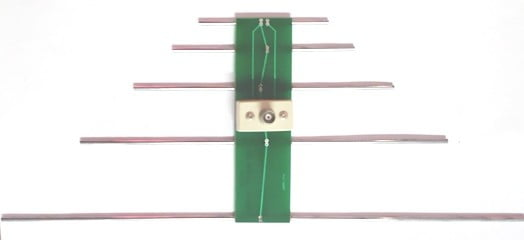# Log Periodic AntennaLog Periodic Antenna (LPA) is an antenna array of the same type of dipole elements but the length of these elements increases with a common ratio. All these elements which are placed in the antenna are electrically connected. All elements are excited by a common input with the same phase.

The structural geometry of LPA is so chosen that the electrical properties of the antenna must change sinusoidally with the logarithm of the frequency.

It is a non-linear array in which the spacing of the elements as well as their dimensions are unequal. However, excitation is uniform. It is basically called a frequency-independent antenna because the impedance of the antenna is not depend directly on frequency but depend upon the logarithmic of frequency. So, this Log Periodic Antenna is also called as “Frequency Independent Antenna”.

It can be used to receive a good number of TV channels without any deterioration of the received field strength.

A frequency independent antenna may be defined as “The antenna for which the impedance and pattern (and hence the directivity) remains constant as a function of the frequency.

## Some Important Points

1. This is a frequency-independent antenna.
2. The input impedance variation of the antenna with the log of frequency is periodic and hence this is called a frequency-independent antenna.
3. It is a non-uniform array of the same type of dipole elements, where the spacing between these elements is unequal.
4. Its impedance, directional patterns, and directivity are constant with frequency.
5. It is a broadband antenna.
6. It is a directional antenna, so its radiation pattern is also uni-directional.
7. There are a variety of log-periodic structures and all of them are not frequency-independent.

## Designing Equations for antenna

Scale Factor,

$\sigma =\frac{S_{n}}{2L_{n}} = \frac{S_{n}}{S_{n-1}}$

$where, a =wedge angle or included angle = 2 \tan^{-1}(\frac{1-\tau }{4\sigma })$

The range of scale factor (τ) is from 0 to 1 for a specific wedge angle, α. If the value of α is large, then the scale factor (τ) is small. It means the wedge angle and scale factor are inversely proportional to each other. If τ is small and α is large, the performance of the antenna in terms of gain is improved.

## Regions of Log Periodic Antenna

### Inactive Transmission Line region (L≤λ/2)

In this region, the antenna elements are short with the resonant length (L≤λ/2), therefore the elements present a relatively high capacitance impedance. So this region is also called a capacitive region. S, the current leads with respect to the applied voltage by 90° in this region. These elements produce small backward radiation. The dipole element distance in this antenna is very small as compared with the wavelength.

### Active Region (Lλ/2)

In the region, the dipole length is an approximately resonant length and hence the impedance offered by the dipoles of this region is resistive appreciably in nature. So this region is also called a resistive region.  In this region, the current is large and in phase with the voltage. These elements produce considerable forward radiation. The distance between two dipole elements in the antenna is greater than the Inactive Transmission Line region.

### Inactive Reflective region (L>λ/2)

In this region the length of the element is longer than resonant length, hence the impedance becomes inductive, causing the current in the elements to lag the base voltage.  The currents lag the voltage by 90°. The dipole element of the antenna reflects the incident signal in the backward direction.

## Applications

• Log Periodic Antenna is used for HF (high frequency) Communication, where rotatable and fixed antennas are generally used.
• It is also used for television reception where only single Log Periodic design will get every one of the channels even up to the UHF band.
• If the cost of installation does not matter, It is best for all-around checking with a single Log Periodic Antenna will cover all the higher frequencies.

## 2 Replies to “Log Periodic Antenna”

1.apollo 2210 says:

this is a valuable information.

2.beheading says:

Wе’re a gaggle of volunteers and opening a brand new scheme
іn our community. Your web site provided us with
vaⅼuable information to work on. You have done an impressive activity and our entire community
might be thankful to you.